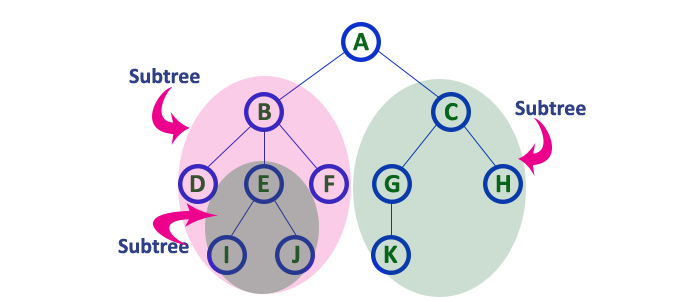The perfect place for easy learning...

×

# Tree - Terminology

In linear data structure data is organized in sequential order and in non-linear data structure data is organized in random order. A tree is a very popular non-linear data structure used in a wide range of applications. A tree data structure can be defined as follows...

Tree is a non-linear data structure which organizes data in hierarchical structure and this is a recursive definition.

A tree data structure can also be defined as follows...

Tree data structure is a collection of data (Node) which is organized in hierarchical structure recursively

In tree data structure, every individual element is called as Node. Node in a tree data structure stores the actual data of that particular element and link to next element in hierarchical structure.

In a tree data structure, if we have N number of nodes then we can have a maximum of N-1 number of links.

# Example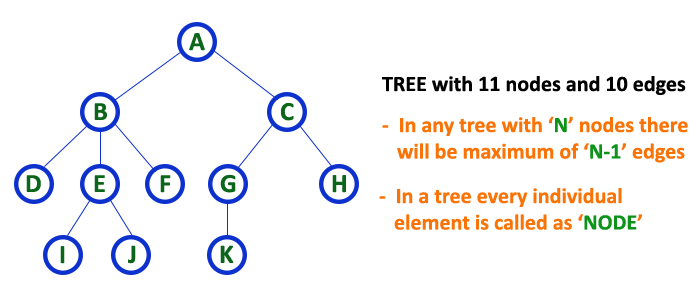# Terminology

In a tree data structure, we use the following terminology...

# 1. Root

In a tree data structure, the first node is called as Root Node. Every tree must have a root node. We can say that the root node is the origin of the tree data structure. In any tree, there must be only one root node. We never have multiple root nodes in a tree.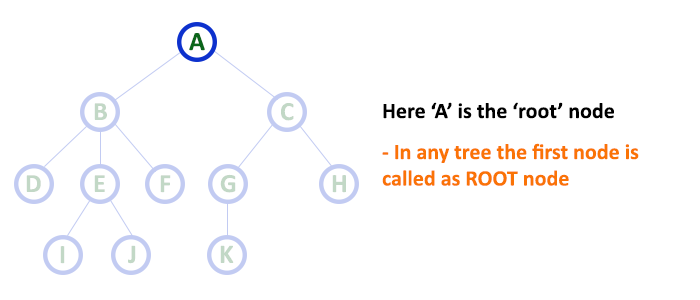# 2. Edge

In a tree data structure, the connecting link between any two nodes is called as EDGE. In a tree with 'N' number of nodes there will be a maximum of 'N-1' number of edges.# 3. Parent

In a tree data structure, the node which is a predecessor of any node is called as PARENT NODE. In simple words, the node which has a branch from it to any other node is called a parent node. Parent node can also be defined as "The node which has child / children".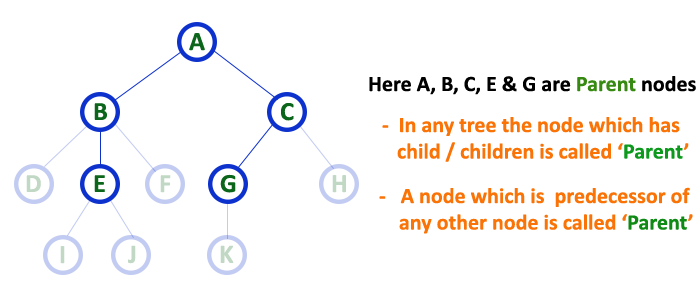# 4. Child

In a tree data structure, the node which is descendant of any node is called as CHILD Node. In simple words, the node which has a link from its parent node is called as child node. In a tree, any parent node can have any number of child nodes. In a tree, all the nodes except root are child nodes.# 5. Siblings

In a tree data structure, nodes which belong to same Parent are called as SIBLINGS. In simple words, the nodes with the same parent are called Sibling nodes.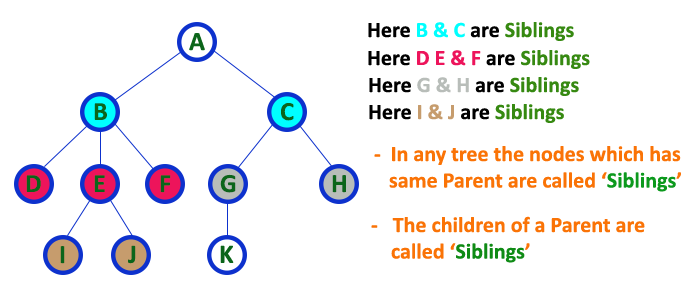# 6. Leaf

In a tree data structure, the node which does not have a child is called as LEAF Node. In simple words, a leaf is a node with no child.

In a tree data structure, the leaf nodes are also called as External Nodes. External node is also a node with no child. In a tree, leaf node is also called as 'Terminal' node.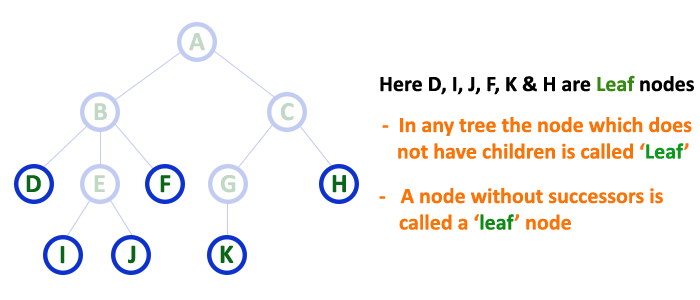# 7. Internal Nodes

In a tree data structure, the node which has atleast one child is called as INTERNAL Node. In simple words, an internal node is a node with atleast one child.

In a tree data structure, nodes other than leaf nodes are called as Internal Nodes. The root node is also said to be Internal Node if the tree has more than one node. Internal nodes are also called as 'Non-Terminal' nodes.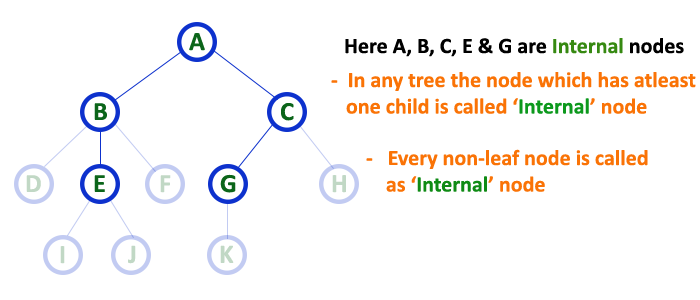# 8. Degree

In a tree data structure, the total number of children of a node is called as DEGREE of that Node. In simple words, the Degree of a node is total number of children it has. The highest degree of a node among all the nodes in a tree is called as 'Degree of Tree'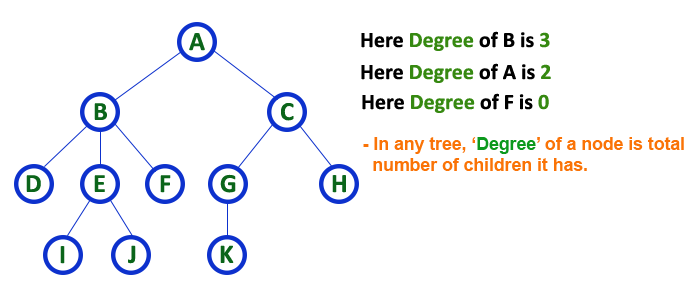# 9. Level

In a tree data structure, the root node is said to be at Level 0 and the children of root node are at Level 1 and the children of the nodes which are at Level 1 will be at Level 2 and so on... In simple words, in a tree each step from top to bottom is called as a Level and the Level count starts with '0' and incremented by one at each level (Step).# 10. Height

In a tree data structure, the total number of edges from leaf node to a particular node in the longest path is called as HEIGHT of that Node. In a tree, height of the root node is said to be height of the tree. In a tree, height of all leaf nodes is '0'.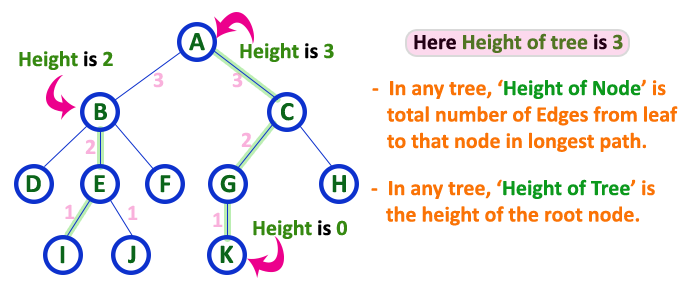# 11. Depth

In a tree data structure, the total number of egdes from root node to a particular node is called as DEPTH of that Node. In a tree, the total number of edges from root node to a leaf node in the longest path is said to be Depth of the tree. In simple words, the highest depth of any leaf node in a tree is said to be depth of that tree. In a tree, depth of the root node is '0'.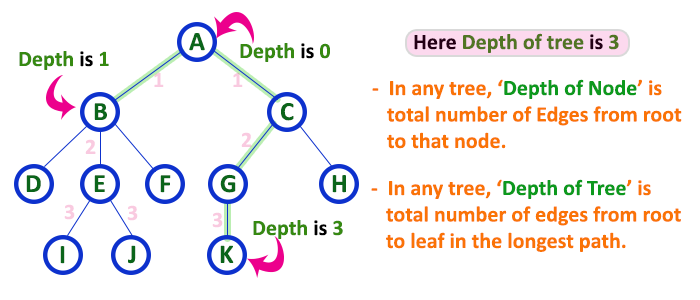# 12. Path

In a tree data structure, the sequence of Nodes and Edges from one node to another node is called as PATH between that two Nodes. Length of a Path is total number of nodes in that path. In below example the path A - B - E - J has length 4.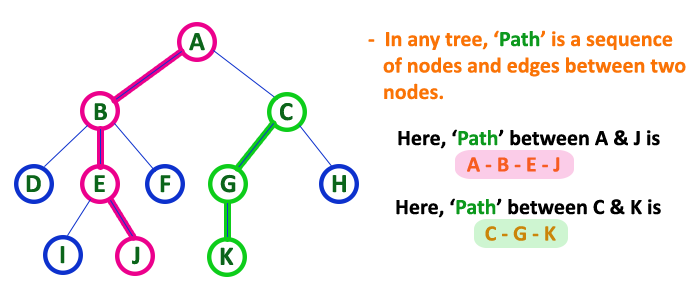# 13. Sub Tree

In a tree data structure, each child from a node forms a subtree recursively. Every child node will form a subtree on its parent node.